# Node with the maximum value in a Binary Search Tree

## Introduction

### Binary search tree (BST)

Before jumping to the question, let’s understand what a Binary search tree is:

A Binary search tree (BST)  is a type of binary tree in which the nodes are arranged in a specific order. It is also known as an ordered binary tree.

Properties of a Binary search tree (BST):

• The value of all the nodes in the left subtree of a node is smaller than the node’s value.
• The value of all the nodes in the right subtree of a node is greater than the node’s value.
• All the left subtrees and right subtrees of the root node must follow the above properties.

Example: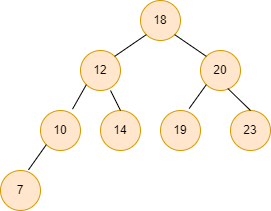### Problem statement

We are given a Binary search tree. Our task is to find the node with the maximum value in the Binary search tree.

Example:

Input1: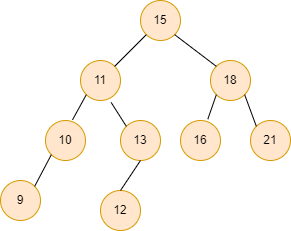Output1:  21    // maximum value in the Binary search tree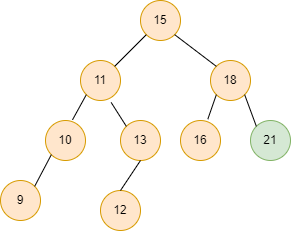Input2: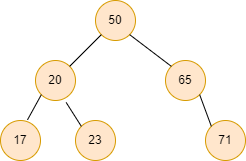Output2: 71    // maximum value in the Binary search tree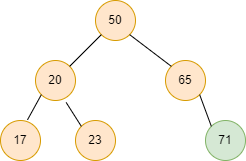## Approach

Finding the maximum value in the Binary search tree is a simple operation because of its ordered structure.

In a Binary search tree (BST), the left child of each node must be less than its parent node, and the right child must be greater than its parent.

So, if we consider the root node of the Binary search tree (BST), the left subtree of the binary search tree must have nodes with values less than or equal to the root node, and the right subtree must have nodes with values greater than the root node.

So, the node with the maximum value in the Binary search tree is the rightmost node of the tree.

Algorithm:

• Declare a curr pointer pointing to the root of the Binary search tree.
• Run a while loop till curr -> right != NULL  and do curr = curr -> right inside the while loop.
• After the completion of the loop, print curr -> data, which is the maximum value in the Binary search tree.

Let’s understand the above algorithm with an example:

Input:Steps:

Initially, the curr pointer points to the root of the Binary search tree.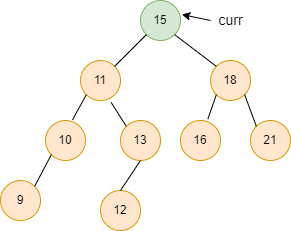Now, curr->right!=NULL, so we move the curr pointer to the right node, curr = curr -> right.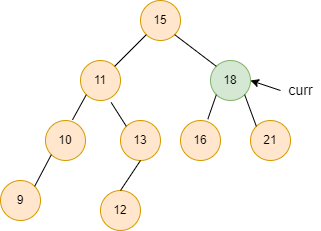Similarly, curr = curr -> right.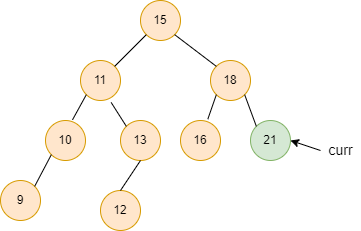Here curr -> right = NULL, print the curr -> data, which is the maximum value in the Binary search tree.

## Code in C++

``````#include <bits/stdc++.h>
using namespace std;

struct Node {
int data;
struct Node* left;
struct Node* right;
};

Node* new_node(int x)
{
Node* freshnode = new Node();
freshnode->data = x;
freshnode->left = NULL;
freshnode->right = NULL;

return (freshnode);
}
Node* insert_bst( Node* node, int data)
{

if (node == NULL)
return (new_node(data));
else
{
if (data <= node->data)
node->left = insert_bst(node->left, data);
else
node->right = insert_bst(node->right, data);

return node;
}
}

void max_val(Node* root)
{
Node* curr = root;

while (curr->right != NULL)
curr = curr->right;

cout << curr-> data << endl;
}

int main()
{
Node* root = NULL;
root = insert_bst(root, 15);
insert_bst(root, 11);
insert_bst(root, 18);
insert_bst(root, 10);
insert_bst(root, 13);
insert_bst(root, 16);
insert_bst(root, 21);
insert_bst(root, 9);
insert_bst(root, 12);

max_val(root);

return 0;
}``````

Output

``21``

### Complexity Analysis

Time complexity: O(n), where n is the number of nodes in the given tree as in the worst case, we have to traverse every node of the tree.

Space complexity: O(1) requires constant extra space.

Q1. In a binary search tree, how will you find the minimum element?

Ans: In a binary search tree, iterating through the tree to the leftmost leaf of the root will give the minimum element because all the elements lesser than a given node are towards the left.

Q2. What are the minimum and the maximum number of nodes in a complete binary tree of height h?

Ans: 2h+1 – 1 is the maximum number of nodes in a full binary tree of height "h." 2h+1 is the minimum number of nodes in a full binary tree of height "h."

Q3. What is the maximum height of a binary tree?

Ans: A node in a binary tree can only have two children. When there are n nodes in a binary tree, the maximum height is n-1.

Q4. How do you find max heap in data structure?

Ans: Remove the root node first.

Step 2: Move the last element from the previous level to the root.

Step 3: Compare this child node's value to its parent’s.

Step 4: If the parent's value is less than the child's, swap them.

## Key Takeaways

So, this article discussed the binary search tree, its properties and how to find the maximum value in a binary search tree with its complete explanation with examples and its C++ code.

If you are a beginner, interested in coding and want to learn DSA, you can look for our guided path for DSA, which is free!